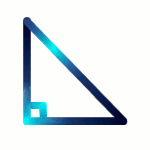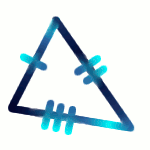# Two-Dimensional Shapes

Two-dimensional Shapes have dimensions in only 2 directions - a width and a length.

Plane Shapes - Same as 2-dimensional shapes; The word plane means flat because these shapes are on flat surfaces

Polygons - 2D shapes with straight sides e.g. triangle, rectangle

Regular Polygons - Polygons with all sides of equal length e.g. equilateral triangle and square

## Types of Polygons

 NUMBER OF SIDES NAME OF POLYGON 3 triangle 4 quadrilateral 5 pentagon 6 hexagon 7 heptagon 8 octagon 9 nonagon 10 decagon 11 undecagonun- = 1deca- = 10 12 dodecagondo- = 2deca- = 10

## Did You Know That...?

Most names for 2D shapes and 3D solids come from the Greek language.

Many 2D shapes have names ending in -gon. Many 3D solids have names ending in -hedron.

Hept- is Greek for "seven". Sept- is Latin for "seven".

## Types of Triangles

 DIAGRAM NAME OF TRIANGLE PROPERTIESRight-angled triangle Has one angle of 90°Longest side is called the hypotenuseEquilateral triangle 3 sides are equal3 angles are equalEach angle is 60°Isosceles triangle 2 sides are equal2 angles are equalScalene triangle No sides are equalNo angles are equal

## Types of Quadrilaterals

 PARALLELOGRAMOpposite sides parallel Opposite sides equal Opposite angles equal Diagonals bisect each other RHOMBUSOpposite sides parallel Opposite sides equal Opposite angles equal All sides equal Diagonals equal Diagonals bisect each other Diagonals meet at right angles Diagonals bisect the angles at the vertices (corners) RECTANGLEOpposite sides parallel Opposite sides equal Opposite angles equal All sides equal Diagonals equal Diagonals bisect each other SQUAREOpposite sides parallel Opposite sides equal Opposite angles equal All sides equal All angles equal Diagonals equal Diagonals bisect each other Diagonals meet at right angles Diagonals bisect the angles at the vertices (corners) TRAPEZOIDOpposite sides parallel (one pair only) Diagonals bisect each other KITE2 pairs of sides equal but not opposite sides equal 1 pair of opposite angles equal 1 diagonal bisects the other diagonal Diagonals meet at right angles 1 diagonal bisects one pair of angles

## Questions - What am I?

Q1. I have 3 sides of equal length.

Q2. I have 4 equal sides, my diagonals bisect each other and the angles, and none of my angles are right angles.

Q3. I have 2 pairs of equal sides, my diagonals are not equal, my diagonals meet each other at right angles, and one pair of my opposite angles is equal.

Answers
A1. equilateral triangle
A2. rhombus
A3. kite